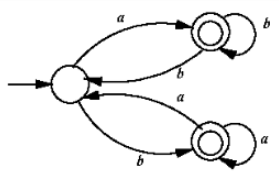October 4, 2023
October 4, 2023
October 4, 2023
###### Programming-for-Output-Problems
October 4, 2023
 Question 11
Which one of the following regular expressions correctly represents the language of the finite automaton given below?A ab*bab* + ba*aba* B (ab*b)*ab* + (ba*a)*ba* C (ab*b + ba*a)* (a* + b*) D (ba*a + ab*b)* (ab* + ba*)
Question 11 Explanation:
Regular expression ab*bab* +ba*aba* does not generate the string “abb” which is accepted by FA hence this is not true.
(ab*b)*ab*+(ba*a)*ba* does not generate the string “abbaa” hence this is also wrong.
(ab*b+ba*a)*(a*+b*) generates epsilon hence this is also wrong.
(ab*b+ba*a)*(ab*+ba*) is the correct regular expression.
GATE 2022 Computer Science and Information Technology (CS)
Question 11 Explanation:
Regular expression ab*bab* +ba*aba* does not generate the string “abb” which is accepted by FA hence this is not true.
(ab*b)*ab*+(ba*a)*ba* does not generate the string “abbaa” hence this is also wrong.
(ab*b+ba*a)*(a*+b*) generates epsilon hence this is also wrong.
(ab*b+ba*a)*(ab*+ba*) is the correct regular expression.
GATE 2022 Computer Science and Information Technology (CS)